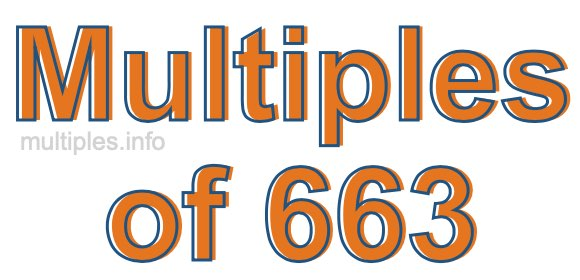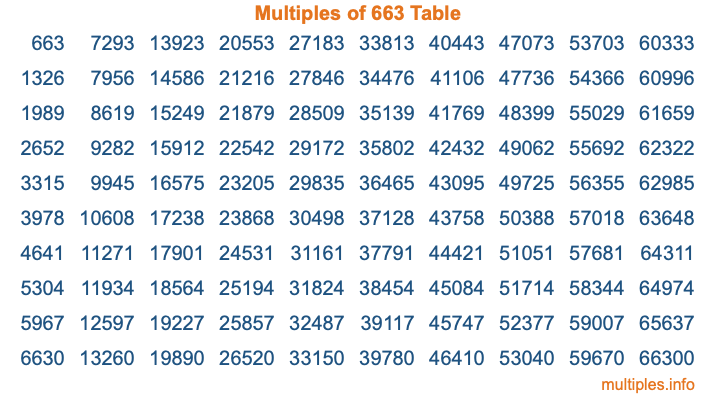Multiples of 663Welcome to the Multiples of 663 page. Here we will first teach you everything you will ever need to know about the multiples of 663, and then give you a study guide summary of everything we taught you to make sure you remember it all. Use this page to look up facts and learn information about the multiples of 663. This page will make you a multiples of six hundred sixty-three expert!

Definition of Multiples of 663
Multiples of 663 are all the numbers that when divided by 663 equal an integer. Each of the multiples of 663 are called a multiple. A multiple of 663 is created by multiplying 663 by an integer.

Therefore, to create a list of multiples of 663, you start with 1 multiplied by 663, then 2 multiplied by 663, then 3 multiplied by 663, and so on for as long as you want. Thus, the list of the first five multiples of 663 is 663, 1326, 1989, 2652, and 3315. To see a larger list of multiples of 663, see the printable image of Multiples of 663 further down on this page. We also have a category where you can choose any nth multiple of 663.

Multiples of 663 Checker
The Multiples of 663 Checker below checks to see if any number of your choice is a multiple of 663. In other words, it checks to see if there is any number (integer) that when multiplied by 663 will equal your number. To do that, we divide your number by 663. If the the quotient is an integer, then your number is a multiple of 663.

Is  a multiple of 663?

Least Common Multiple of 663 and ...
A Least Common Multiple (LCM) is the lowest multiple that two or more numbers have in common. This is also called the smallest common multiple or lowest common multiple and is useful to know when you are adding our subtracting fractions. Enter one or more numbers below (663 is already entered) to find the LCM.

Check out our LCM Calculator if you need more details about the Least Common Multiple or if you need the LCM for different numbers for adding and subtraction fractions.

nth Multiple of 663
As we stated above, 663 is the first multiple of 663, 1326 is the second multiple of 663, 1989 is the third multiple of 663, and so on. Enter a number below to find the nth multiple of 663.

th multiple of 663

Multiples of 663 vs Factors of 663
663 is a multiple of 663 and a factor of 663, but that is where the similarities end. All postive multiples of 663 are 663 or greater than 663. All positive factors of 663 are 663 or less than 663.

Below is the beginning list of multiples of 663 and the factors of 663 so you can compare:

Multiples of 663: 663, 1326, 1989, 2652, 3315, etc.

Factors of 663: 1, 3, 13, 17, 39, 51, 221, 663

As you can see, the multiples of 663 are all the numbers that you can divide by 663 to get a whole number. The factors of 663, on the other hand, are all the whole numbers that you can multiply by another whole number to get 663.

It's also interesting to note that if a number (x) is a factor of 663, then 663 will also be a multiple of that number (x).

Multiples of 663 vs Divisors of 663
The divisors of 663 are all the integers that 663 can be divided by evenly. Below is a list of the divisors of 663.

Divisors of 663: 1, 3, 13, 17, 39, 51, 221, 663

The interesting thing to note here is that if you take any multiple of 663 and divide it by a divisor of 663, you will see that the quotient is an integer.

Multiples of 663 Table
Below is an image of the first 100 multiples of 663 in a table. The table is in chronological order, column by column. The first column has the first ten multiples of 663, the second column has the next ten multiples of 663, and so on.The Multiples of 663 Table is also referred to as the 663 Times Table or Times Table of 663. You are welcome to print out our table for your studies.

Negative Multiples of 663
Although not often discussed or needed in math, it is worth mentioning that you can make a list of negative multiples of 663 by multiplying 663 by -1, then by -2, then by -3, and so on, to get the following list of negative multiples of 663:

-663, -1326, -1989, -2652, -3315, etc.

Multiples of 663 Summary
Below is a summary of important Multiples of 663 facts that we have discussed on this page. To retain the knowledge on this page, we recommend that you read through the summary and explain to yourself or a study partner why they hold true.

There are an infinite number of multiples of 663.

A multiple of 663 divided by 663 will equal a whole number.

663 divided by a factor of 663 equals a divisor of 663.

The nth multiple of 663 is n times 663.

The largest factor of 663 is equal to the first positive multiple of 663.

663 is a multiple of every factor of 663.

663 is a multiple of 663.

A multiple of 663 divided by a divisor of 663 equals an integer.

663 divided by a divisor of 663 equals a factor of 663.

Any integer times 663 will equal a multiple of 663.

Multiples of a Number
Here you can get the multiples of another number, all with the same attention to detail as we did for multiples of 663 on this page.

Multiples of
Multiples of 664
Did you find our page about multiples of six hundred sixty-three educational? Do you want more knowledge? Check out the multiples of the next number on our list!